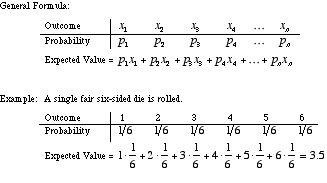index: click on a letter A B C D E F G H I J K L M N O P Q R S T U V W X Y Z A to Z index index: subject areas numbers & symbols sets, logic, proofs geometry algebra trigonometry advanced algebra & pre-calculus calculus advanced topics probability & statistics real world applications multimedia entrieswww.mathwords.com about mathwords website feedback

 Expected Value Mean of a Random Variable A quantity equal to the average result of an experiment after a large number of trials. For example, if a fair 6-sided die is rolled, the expected value of the number rolled is 3.5. This is a correct interpretation even though it is impossible to roll a 3.5 on a 6-sided die. This sort of thing often occurs with expected values.See also Weighted average